Hello, Guest!
 Instructional Focus DocumentGeometry
 TITLE : Unit 05: Relationships of Right Triangles, including Trigonometry SUGGESTED DURATION : 22 days

#### Unit Overview

Introduction
This unit bundles student expectations that address constructions of right triangles and right triangle relationships including the Pythagorean Theorem, Pythagorean triples, special right triangles, and trigonometric ratios. Concepts are incorporated into both mathematical and real-world problem situations. According to the Texas Education Agency, mathematical process standards including application, tools and techniques, communication, representations, relationships, and justifications should be integrated (when applicable) with content knowledge and skills so that students are prepared to use mathematics in everyday life, society, and the workplace.

Prior to this Unit
In Grade 8, students were introduced to the Pythagorean Theorem and its converse and applications of these in problem situations. In Geometry Unit 04, students studied triangles, including triangle relationships, congruent triangles, similar triangles, and special segments of triangles.

During this Unit
Students concretely explore and prove the Pythagorean Theorem and its converse. Students examine patterns of given Pythagorean triples to discover other Pythagorean triples. Students use constructions of right triangles and their altitudes to investigate and analyze geometric relationships, including geometric mean. Students explore geometric relationships in special right triangles (30°-60°-90° and 45°-45°-90°) and similar triangles. Students develop right triangle trigonometry and trigonometric ratios to determine side lengths and angle measures in right triangles. Students apply all right triangle geometric relationships to solve both mathematical and real-world problem situations.

Other considerations: Reference the Mathematics COVID-19 Gap Implementation Tool HS Geometry

After this Unit
In Units 06 and 08, students will continue to use the concepts of right triangles to investigate and solve problems involving properties and measurement of figures. In subsequent courses in mathematics, these concepts will continue to be applied to problem situations involving triangles.

This unit is supporting the development of Texas College Career Readiness Standards (TxCCRS): I. Numeric Reasoning B1; II. Algebraic Reasoning A1, D1, D2; III. Geometric and Spatial Reasoning A2, A3, B1, B2, C1, D3; V. Statistical Reasoning A1, C2; VII. Problem Solving and Reasoning A1, A2, A3, A4, A5, B1, C1, D1, D2; VIII. Communication and Representation A1, A2, A3, B1, B2, C1, C2, C3; IX. Connections A1, A2, B1, B2, B3.

Research
According to the National Council of Teachers of Mathematics (2000), all students in grades 9 – 12 should explore relationships (including congruence and similarity) in two-dimensional geometric figures, make and test conjectures about two-dimensional geometric figures, and solve problems involving two-dimensional geometric figures. According to the National Council of Teachers of Mathematics (2012), using diagrams and constructions to interpret and communicate geometric relationships is essential in geometry. Using definitions of figures to characterize figures in terms of their properties is another essential in geometry. In geometry, the “proving process involves working with diagrams, variation and invariance, conjectures, and definitions.” (p. 92)

National Council of Teachers of Mathematics. (2000). Principles and standards for school mathematics. Reston, VA: National Council of Teachers of Mathematics, Inc.
National Council of Teachers of Mathematics. (2012). Developing essential understanding of Geometry for Teaching Mathematics in Grades 9 – 12. Reston, VA: National Council of Teachers of Mathematics, Inc.
Texas Education Agency & Texas Higher Education Coordinating Board. (2009). Texas college and career readiness standards. Retrieved from http://www.thecb.state.tx.us/index.cfm?objectid=E21AB9B0-2633-11E8-BC500050560100A9

 Geometric, spatial, and measurement reasoning are foundational to visualizing, analyzing, and applying relationships within and between scale, shapes, quantities, and spatial relations in everyday life. Why is developing geometric, spatial, and measurement reasoning essential? How does geometric, spatial, and measurement reasoning affect how one sees and works in the world? Logical reasoning can be used to make sense of claims, determine their validity, and construct and communicate arguments. Why is developing logical reasoning in mathematics important and how does this reasoning influence decision making in everyday life? What elements of logical reasoning influence the truth of a statement? How is logical reasoning used to uncover truths and/or make sense of, construct, and determine the validity of arguments and claims?
Unit Understandings
and Questions
Overarching Concepts
and Unit Concepts
Performance Assessment(s)
• Understanding and working with variance and invariance within geometry builds flexible algebraic and geometric reasoning and deepens understanding of intrinsic properties of geometric relationships.
• What invariant (unchanging) and variant (changing) relationships exist within right triangles?
• What invariant (unchanging) and variant (changing) relationships exist within side lengths and angle measures in triangles?
• How does examining variance and invariance lead to new conjectures and theorems about right triangle relationships?
• Deductive reasoning can be used to determine the validity of a conditional statement and its related statements and conjectures about geometric relationships in order to support or refute mathematical claims through the process of proving.
• How is deductive reasoning used to understand, prove, and apply geometric conjectures about right triangle relationships?
• side lengths of right triangles?
• angle measures of right triangles?
• right triangle similarity?
• How can the converse of theorems and postulates be used to solve problem situations about right triangles?
• Accurate representations, models, or diagrams within a geometric system allows for visualizing, illustrating, and analyzing geometric relationships to aid in making and validating conjectures about those geometric relationships and is central to geometric thinking.
• What types of problem situations represent right triangle relationships?
• How can representations and appropriate geometric language be used to effectively communicate and illustrate geometric relationships of right triangles?
• What tools and processes can be used to …
• explore the Pythagorean Theorem and its converse?
• discover Pythagorean triples?
• What conjectures about congruency and similarity can be made and validated by exploring the patterns and properties of right triangles?
• Attributes and quantifiable measures of geometric figures can be generalized to describe, determine, and represent algebraic and geometric relationships and be applied to solve problem situations.
• How can understanding right triangle relationships be applied when solving problem situations?
• How can measurable attributes related to …
• angle measures
• side lengths
• Pythagorean Theorem
… be distinguished and described in order to generalize geometric relationships of right triangles?
• What processes can be used to determine the …
• missing side length
• missing angle measure
• proportional side lengths
… of a right triangle?
• How can Pythagorean triples be used to efficiently determine unknown side lengths in a right triangle?
• Similarity, Proof, and Trigonometry
• Geometric Relationships
• Congruence
• Equivalence
• Perpendicularity
• Similarity
• Proportionality
• Corresponding sides and angles
• Measure relationships
• Formulas
• Geometric Representations
• Two-dimensional figures
• Two-Dimensional Coordinate Systems
• Distance
• Patterns, Operations, and Properties
• Transformations
• Non-rigid transformations
• Triangle Relationships
• Similar triangles
• Right triangles
• Pythagorean Theorem
• Pythagorean triples
• Proof and Congruence
• Deductive Reasoning
• Definitions
• Conjectures
• Theorems
• Proofs
• Geometric Relationships
• Triangle relationships
• Geometric Representations
• Angles
• Sides
• Patterns, Operations, and Properties
• Associated Mathematical Processes
• Application
• Problem Solving Model
• Tools and Techniques
• Communication
• Representations
• Relationships
• Justification
 Assessment information provided within the TEKS Resource System are examples that may, or may not, be used by your child’s teacher. In accordance with section 26.006 (2) of the Texas Education Code, "A parent is entitled to review each test administered to the parent’s child after the test is administered." For more information regarding assessments administered to your child, please visit with your child’s teacher.

 Geometric, spatial, and measurement reasoning are foundational to visualizing, analyzing, and applying relationships within and between scale, shapes, quantities, and spatial relations in everyday life. Why is developing geometric, spatial, and measurement reasoning essential? How does geometric, spatial, and measurement reasoning affect how one sees and works in the world? Logical reasoning can be used to make sense of claims, determine their validity, and construct and communicate arguments. Why is developing logical reasoning in mathematics important and how does this reasoning influence decision making in everyday life? What elements of logical reasoning influence the truth of a statement? How is logical reasoning used to uncover truths and/or make sense of, construct, and determine the validity of arguments and claims?
Unit Understandings
and Questions
Overarching Concepts
and Unit Concepts
Performance Assessment(s)
• Understanding and working with variance and invariance within geometry builds flexible algebraic and geometric reasoning and deepens understanding of intrinsic properties of geometric relationships.
• What invariant (unchanging) and variant (changing) relationships exist within right triangles?
• What invariant (unchanging) and variant (changing) relationships exist within side lengths and angle measures in triangles?
• How does examining variance and invariance lead to new conjectures and theorems about triangle relationships?
• Deductive reasoning can be used to determine the validity of a conditional statement and its related statements and conjectures about geometric relationships in order to support or refute mathematical claims through the process of proving.
• How is deductive reasoning used to understand, prove, and apply geometric conjectures about triangle relationships?
• relationships in right triangles?
• side lengths of triangles?
• angle measures of triangles?
• the relationship of special triangle segments?
• triangle similarity?
• How can the converse of theorems and postulates be used to solve problem situations about right triangles?
• Accurate representations, models, or diagrams within a geometric system allows for visualizing, illustrating, and analyzing geometric relationships to aid in making and validating conjectures about those geometric relationships and is central to geometric thinking.
• What types of problem situations represent right triangle relationships?
• How can representations and appropriate geometric language be used to effectively communicate and illustrate geometric relationships about triangles?
• What tools and processes can be used to explore the Pythagorean Theorem and its converse?
• How can constructions be used to make and validate conjectures about geometric relationships of triangles?
• What conjectures about similarity can be made and validated by exploring the patterns and properties of triangles?
• Attributes and quantifiable measures of geometric figures can be generalized to describe, determine, and represent algebraic and geometric relationships and be applied to solve problem situations.
• How can understanding right triangle relationships be applied when solving problem situations?
• How can measurable attributes related to …
• angle measures
• side lengths
• Pythagorean Theorem
• Pythagorean triples
… be distinguished and described in order to generalize geometric relationships of triangles?
• What processes can be used to determine the …
• geometric mean
• missing side length
• missing angle measure
• proportional side lengths
… of a right triangle?
• How can Pythagorean triples be used to efficiently determine unknown side lengths in a right triangle?
• Similarity, Proof, and Trigonometry
• Geometric Relationships
• Equivalence
• Perpendicularity
• Similarity
• Proportionality
• Corresponding sides and angles
• Measure relationships
• Formulas
• Geometric Representations
• Two-dimensional figures
• Patterns, Operations, and Properties
• Transformations
• Non-rigid transformations
• Triangle Relationships
• Similar triangles
• Special right triangles
• Pythagorean Theorem
• Pythagorean triples
• Logical Arguments and Constructions; Similarity, Proof, and Trigonometry
• Constructions
• Congruent segments
• Congruent angles
• Segment bisector
• Angle bisector
• Perpendicular lines
• Perpendicular bisector
• Deductive Reasoning
• Definitions
• Conjectures
• Theorems
• Geometric Relationships
• Perpendicularity
• Triangle relationships
• Geometric Representations
• Angles
• Sides
• Segments
• Triangles
• Patterns, Operations, and Properties
• Associated Mathematical Processes
• Application
• Problem Solving Model
• Tools and Techniques
• Communication
• Representations
• Relationships
• Justification
 Assessment information provided within the TEKS Resource System are examples that may, or may not, be used by your child’s teacher. In accordance with section 26.006 (2) of the Texas Education Code, "A parent is entitled to review each test administered to the parent’s child after the test is administered." For more information regarding assessments administered to your child, please visit with your child’s teacher.

 Geometric, spatial, and measurement reasoning are foundational to visualizing, analyzing, and applying relationships within and between scale, shapes, quantities, and spatial relations in everyday life. Why is developing geometric, spatial, and measurement reasoning essential? How does geometric, spatial, and measurement reasoning affect how one sees and works in the world?
Unit Understandings
and Questions
Overarching Concepts
and Unit Concepts
Performance Assessment(s)
• Understanding and working with variance and invariance within geometry builds flexible algebraic and geometric reasoning and deepens understanding of intrinsic properties of geometric relationships.
• What invariant (unchanging) and variant (changing) relationships exist within right triangles?
• What invariant (unchanging) and variant (changing) relationships exist within side and angle relationships in right triangles?
• How does examining variance and invariance lead to new conjectures about right triangle relationships?
• Accurate representations, models, or diagrams within a geometric system allows for visualizing, illustrating, and analyzing geometric relationships to aid in making and validating conjectures about those geometric relationships and is central to geometric thinking.
• What types of problem situations represent right triangle trigonometry?
• How can representations and appropriate geometric language be used to effectively communicate and illustrate geometric relationships about right triangle trigonometry?
• What tools and processes can be used to …
• explore the Pythagorean Theorem and its converse?
• discover Pythagorean triples?
• develop right triangle trigonometry?
• Attributes and quantifiable measures of geometric figures can be generalized to describe, determine, and represent algebraic and geometric relationships and be applied to solve problem situations.
• How can understanding right triangle trigonometry be applied when solving problem situations?
• How can measurable attributes related to …
• angle measures
• side lengths
… be distinguished and described in order to generalize geometric relationships of right triangles?
• What processes can be used to determine the …
• trigonometric ratios in right triangles?
• missing side length of a right triangle?
• missing angle measure of right triangles?
• Similarity, Proof, and Trigonometry
• Geometric Relationships
• Equivalence
• Perpendicularity
• Measure relationships
• Formulas
• Geometric Representations
• Two-dimensional figures
• Patterns, Operations, and Properties
• Triangle Relationships
• Right triangles
• Special right triangles
• Trigonometric ratios
• Pythagorean Theorem
• Pythagorean triples
• Associated Mathematical Processes
• Application
• Problem Solving Model
• Tools and Techniques
• Communication
• Representations
• Relationships
• Justification
 Assessment information provided within the TEKS Resource System are examples that may, or may not, be used by your child’s teacher. In accordance with section 26.006 (2) of the Texas Education Code, "A parent is entitled to review each test administered to the parent’s child after the test is administered." For more information regarding assessments administered to your child, please visit with your child’s teacher.

#### MISCONCEPTIONS / UNDERDEVELOPED CONCEPTS

Misconceptions:

• Some students may not correctly identify which side lengths should be used for the legs (a and b) and the hypotenuse (c) in the Pythagorean Theorem.
• Some students may not use the correct order of operations when applying or solving an equation with the Pythagorean Theorem.
• Although some students may identify a Pythagorean Triple, such as 3, 4, and 5, the students may over-generalize its use to situations where the triple does not apply, such as where the legs of a right triangle are 3 and 5 (instead of 3 and 4).
• Some students may have trouble identifying the adjacent leg and opposite leg for a given acute angle in a right triangle.
• Some students may confuse which trigonometric function is appropriate for the given situation.
• Some students may confuse when to use a trigonometric function (such as sine) and its inverse (sin-1).
• Some students may not correctly identify which angle is the angle of elevation or angle of depression.
• Some students may not correctly apply the special right triangle relationships if the side length opposite the 60o angle or hypotenuse is given rather than the side length opposite the 30° angle.

Underdeveloped Concepts:

• Although some students may know how to work the problem, they may have trouble sketching and labeling the figure appropriately.
• Although some students may correctly use a calculator to evaluate trigonometric functions, the students may get strange or erroneous answers, if the MODE of the calculator is set in RADIANS instead of DEGREES.
• Although some students may know how to use the Pythagorean Theorem, they may have trouble simplifying any radicals produced when solving for a side length.

#### Unit Vocabulary

• 30°–60°–90° right triangle relationships – the hypotenuse is twice as long as the shorter leg, and the longer leg istimes as long as the shorter leg
• 45°–45°–90° right triangle relationships – the legs are equal in measure and the hypotenuse istimes as long as a leg
• Altitude of a triangle – a line segment drawn from any vertex of a triangle perpendicular to the opposite side
• Angle bisector – line, ray, or segment that divides an angle into two congruent angles
• Congruent angles – angles whose angle measurements are equal
• Congruent segments – line segments whose lengths are equal
• Corresponding angles – angles in two figures whose relative position is the same
• Geometric construction – construction of accurate representations of lengths, angles, and geometric figures using only a straight edge and compass
• Geometric mean – a positive number x such that, so x2 = ab and x =• Hypotenuse of a right triangle – the longest side of a right triangle, the side opposite the right angle
• Perpendicular bisector of a line segment – line, ray, or segment that divides a line segment into two congruent segments and forms a 90° angle at the point of intersection
• Perpendicular lines – lines that intersect at a 90° angle to form right angles
• Proportional sides – corresponding side lengths form equivalent ratios
• Pythagorean triples – three positive integers, a, b, and c, such that a2 + b2 = c2, triples can be generated by multiplying a given Pythagorean triple by any positive integer
• Segment bisector – point, line, ray, segment, or point that divides a line segment into two congruent segments
• Similar figures – shapes whose angles are congruent and side lengths are proportional (equal scale factor)
• Trigonometric ratios – a ratio of the measures of two sides of a right triangle based on their position in relation to an acute angle in the right triangle

Related Vocabulary:

 Angle of depression Angle of elevation Converse of Pythagorean Theorem Cosine Equilateral triangle Hypotenuse Isosceles right triangle Leg adjacent Leg of a right triangle Leg opposite Pythagorean Theorem Right triangle Sine Special right triangle Tangent
Unit Assessment Items System Resources Other Resources

Show this message:

Unit Assessment Items that have been published by your district may be accessed through Search All Components in the District Resources tab. Assessment items may also be found using the Assessment Center if your district has granted access to that tool.

System Resources may be accessed through Search All Components in the District Resources Tab.

Texas Higher Education Coordinating Board – Texas College and Career Readiness Standards

Texas Education Agency – Mathematics Curriculum

Texas Education Agency – STAAR Mathematics Resources

Texas Education Agency Texas Gateway – Revised Mathematics TEKS: Vertical Alignment Charts

Texas Education Agency Texas Gateway – Mathematics TEKS: Supporting Information

Texas Education Agency Texas Gateway – Interactive Mathematics Glossary

Texas Education Agency Texas Gateway – Resources Aligned to Geometry Mathematics TEKS

Texas Instruments – Graphing Calculator Tutorials

TAUGHT DIRECTLY TEKS

TEKS intended to be explicitly taught in this unit.

TEKS/SE Legend:

• Knowledge and Skills Statements (TEKS) identified by TEA are in italicized, bolded, black text.
• Student Expectations (TEKS) identified by TEA are in bolded, black text.
• Portions of the Student Expectations (TEKS) that are not included in this unit but are taught in previous or future units are indicated by a strike-through.

Specificity Legend:

• Supporting information / clarifications (specificity) written by TEKS Resource System are in blue text.
• Unit-specific clarifications are in italicized, blue text.
• Information from Texas Education Agency (TEA), Texas College and Career Readiness Standards (TxCCRS), Texas Response to Curriculum Focal Points (TxRCFP) is labeled.
• A Partial Specificity label indicates that a portion of the specificity not aligned to this unit has been removed.
TEKS# SE# TEKS SPECIFICITY
G.1 Mathematical process standards. The student uses mathematical processes to acquire and demonstrate mathematical understanding. The student is expected to:
G.1A Apply mathematics to problems arising in everyday life, society, and the workplace.

Apply

MATHEMATICS TO PROBLEMS ARISING IN EVERYDAY LIFE, SOCIETY, AND THE WORKPLACE

Including, but not limited to:

• Mathematical problem situations within and between disciplines
• Everyday life
• Society
• Workplace

Note(s):

• The mathematical process standards may be applied to all content standards as appropriate.
• TxCCRS:
• VII.D. Problem Solving and Reasoning – Real-world problem solving
• VII.D.1. Interpret results of the mathematical problem in terms of the original real-world situation.
• IX.A. Connections – Connections among the strands of mathematics
• IX.A.1. Connect and use multiple key concepts of mathematics in situations and problems.
• IX.A.2. Connect mathematics to the study of other disciplines.
• IX.B. Connections – Connections of mathematics to nature, real-world situations, and everyday life
• IX.B.1. Use multiple representations to demonstrate links between mathematical and real-world situations.
• IX.B.2. Understand and use appropriate mathematical models in the natural, physical, and social sciences.
• IX.B.3. Know and understand the use of mathematics in a variety of careers and professions.
G.1B Use a problem-solving model that incorporates analyzing given information, formulating a plan or strategy, determining a solution, justifying the solution, and evaluating the problem-solving process and the reasonableness of the solution.

Use

A PROBLEM-SOLVING MODEL THAT INCORPORATES ANALYZING GIVEN INFORMATION, FORMULATING A PLAN OR STRATEGY, DETERMINING A SOLUTION, JUSTIFYING THE SOLUTION, AND EVALUATING THE PROBLEM-SOLVING PROCESS AND THE REASONABLENESS OF THE SOLUTION

Including, but not limited to:

• Problem-solving model
• Analyze given information
• Formulate a plan or strategy
• Determine a solution
• Justify the solution
• Evaluate the problem-solving process and the reasonableness of the solution

Note(s):

• The mathematical process standards may be applied to all content standards as appropriate.
• TxCCRS:
• I.B. Numeric Reasoning – Number sense and number concepts
• I.B.1. Use estimation to check for errors and reasonableness of solutions.
• V.A. Statistical Reasoning – Design a study
• V.A.1. Formulate a statistical question, plan an investigation, and collect data.
• VII.A. Problem Solving and Reasoning – Mathematical problem solving
• VII.A.1. Analyze given information.
• VII.A.2. Formulate a plan or strategy.
• VII.A.3. Determine a solution.
• VII.A.4. Justify the solution.
• VII.A.5. Evaluate the problem-solving process.
• VII.D. Problem Solving and Reasoning – Real-world problem solving
• VII.D.2. Evaluate the problem-solving process.
G.1C Select tools, including real objects, manipulatives, paper and pencil, and technology as appropriate, and techniques, including mental math, estimation, and number sense as appropriate, to solve problems.

Select

TOOLS, INCLUDING REAL OBJECTS, MANIPULATIVES, PAPER AND PENCIL, AND TECHNOLOGY AS APPROPRIATE, AND TECHNIQUES, INCLUDING MENTAL MATH, ESTIMATION, AND NUMBER SENSE AS APPROPRIATE, TO SOLVE PROBLEMS

Including, but not limited to:

• Appropriate selection of tool(s) and techniques to apply in order to solve problems
• Tools
• Real objects
• Manipulatives
• Paper and pencil
• Technology
• Techniques
• Mental math
• Estimation
• Number sense

Note(s):

• The mathematical process standards may be applied to all content standards as appropriate.
• TxCCRS:
• I.B. Numeric Reasoning – Number sense and number concepts
• I.B.1. Use estimation to check for errors and reasonableness of solutions.
• V.C. Statistical Reasoning – Analyze, interpret, and draw conclusions from data
• V.C.2. Analyze relationships between paired data using spreadsheets, graphing calculators, or statistical software.
G.1D Communicate mathematical ideas, reasoning, and their implications using multiple representations, including symbols, diagrams, graphs, and language as appropriate.

Communicate

MATHEMATICAL IDEAS, REASONING, AND THEIR IMPLICATIONS USING MULTIPLE REPRESENTATIONS, INCLUDING SYMBOLS, DIAGRAMS, GRAPHS, AND LANGUAGE AS APPROPRIATE

Including, but not limited to:

• Mathematical ideas, reasoning, and their implications
• Multiple representations, as appropriate
• Symbols
• Diagrams
• Graphs
• Language

Note(s):

• The mathematical process standards may be applied to all content standards as appropriate.
• TxCCRS:
• II.D. Algebraic Reasoning – Representing relationships
• II.D.1. Interpret multiple representations of equations, inequalities, and relationships.
• II.D.2. Convert among multiple representations of equations, inequalities, and relationships.
• VIII.A. Communication and Representation – Language, terms, and symbols of mathematics
• VIII.A.1. Use mathematical symbols, terminology, and notation to represent given and unknown information in a problem.
• VIII.A.2. Use mathematical language to represent and communicate the mathematical concepts in a problem.
• VIII.A.3. Use mathematical language for reasoning, problem solving, making connections, and generalizing.
• VIII.B. Communication and Representation – Interpretation of mathematical work
• VIII.B.1. Model and interpret mathematical ideas and concepts using multiple representations.
• VIII.B.2. Summarize and interpret mathematical information provided orally, visually, or in written form within the given context.
• VIII.C. Communication and Representation – Presentation and representation of mathematical work
• VIII.C.1. Communicate mathematical ideas, reasoning, and their implications using symbols, diagrams, models, graphs, and words.
• VIII.C.2. Create and use representations to organize, record, and communicate mathematical ideas.
• VIII.C.3. Explain, display, or justify mathematical ideas and arguments using precise mathematical language in written or oral communications.
• IX.B. Connections – Connections of mathematics to nature, real-world situations, and everyday life
• IX.B.1. Use multiple representations to demonstrate links between mathematical and real-world situations.
G.1E Create and use representations to organize, record, and communicate mathematical ideas.

Create, Use

REPRESENTATIONS TO ORGANIZE, RECORD, AND COMMUNICATE MATHEMATICAL IDEAS

Including, but not limited to:

• Representations of mathematical ideas
• Organize
• Record
• Communicate
• Evaluation of the effectiveness of representations to ensure clarity of mathematical ideas being communicated
• Appropriate mathematical vocabulary and phrasing when communicating mathematical ideas

Note(s):

• The mathematical process standards may be applied to all content standards as appropriate.
• TxCCRS:
• VIII.B. Communication and Representation – Interpretation of mathematical work
• VIII.B.1. Model and interpret mathematical ideas and concepts using multiple representations.
• VIII.B.2. Summarize and interpret mathematical information provided orally, visually, or in written form within the given context.
• VIII.C. Communication and Representation – Presentation and representation of mathematical work
• VIII.C.1. Communicate mathematical ideas, reasoning, and their implications using symbols, diagrams, models, graphs, and words.
• VIII.C.2. Create and use representations to organize, record, and communicate mathematical ideas.
G.1F Analyze mathematical relationships to connect and communicate mathematical ideas.

Analyze

MATHEMATICAL RELATIONSHIPS TO CONNECT AND COMMUNICATE MATHEMATICAL IDEAS

Including, but not limited to:

• Mathematical relationships
• Connect and communicate mathematical ideas
• Conjectures and generalizations from sets of examples and non-examples, patterns, etc.
• Current knowledge to new learning

Note(s):

• The mathematical process standards may be applied to all content standards as appropriate.
• TxCCRS:
• VII.A. Problem Solving and Reasoning – Mathematical problem solving
• VII.A.1. Analyze given information.
• VIII.A. Communication and Representation – Language, terms, and symbols of mathematics
• VIII.A.1. Use mathematical symbols, terminology, and notation to represent given and unknown information in a problem.
• VIII.A.2. Use mathematical language to represent and communicate the mathematical concepts in a problem.
• VIII.A.3. Use mathematical language for reasoning, problem solving, making connections, and generalizing.
•  VIII.B. Communication and Representation – Interpretation of mathematical work
• VIII.B.1. Model and interpret mathematical ideas and concepts using multiple representations.
• VIII.C. Communication and Representation – Presentation and representation of mathematical work
• VIII.C.1. Communicate mathematical ideas, reasoning, and their implications using symbols, diagrams, models, graphs, and words.
• VIII.C.2. Create and use representations to organize, record, and communicate mathematical ideas.
• VIII.C.3. Explain, display, or justify mathematical ideas and arguments using precise mathematical language in written or oral communications.
• IX.A. Connections – Connections among the strands of mathematics
• IX.A.1. Connect and use multiple key concepts of mathematics in situations and problems.
• IX.A.2. Connect mathematics to the study of other disciplines.
G.1G Display, explain, and justify mathematical ideas and arguments using precise mathematical language in written or oral communication.

Display, Explain, Justify

MATHEMATICAL IDEAS AND ARGUMENTS USING PRECISE MATHEMATICAL LANGUAGE IN WRITTEN OR ORAL COMMUNICATION

Including, but not limited to:

• Mathematical ideas and arguments
• Validation of conclusions
• Displays to make work visible to others
• Diagrams, visual aids, written work, etc.
• Explanations and justifications
• Precise mathematical language in written or oral communication

Note(s):

• The mathematical process standards may be applied to all content standards as appropriate.
• TxCCRS:
• VII.A. Problem Solving and Reasoning – Mathematical problem solving
• VII.A.4. Justify the solution.
• VII.B. Problem Solving and Reasoning – Proportional reasoning
• VII.B.1. Use proportional reasoning to solve problems that require fractions, ratios, percentages, decimals, and proportions in a variety of contexts using multiple representations.
• VII.C. Problem Solving and Reasoning – Logical reasoning
• VII.C.1. Develop and evaluate convincing arguments.
• VIII.A. Communication and Representation – Language, terms, and symbols of mathematics
• VIII.A.3. Use mathematical language for reasoning, problem solving, making connections, and generalizing.
• VIII.B. Communication and Representation – Interpretation of mathematical work
• VIII.B.1. Model and interpret mathematical ideas and concepts using multiple representations.
• VIII.B.2. Summarize and interpret mathematical information provided orally, visually, or in written form within the given context.
• VIII.C. Communication and Representation – Presentation and representation of mathematical work
• VIII. C.3. Explain, display, or justify mathematical ideas and arguments using precise mathematical language in written or oral communications.
G.5 Logical argument and constructions. The student uses constructions to validate conjectures about geometric figures. The student is expected to:
G.5B

Construct congruent segments, congruent angles, a segment bisector, an angle bisector, perpendicular lines, the perpendicular bisector of a line segment, and a line parallel to a given line through a point not on a line using a compass and a straightedge.

Construct

CONGRUENT SEGMENTS, CONGRUENT ANGLES, A SEGMENT BISECTOR, AN ANGLE BISECTOR, PERPENDICULAR LINES, AND THE PERPENDICULAR BISECTOR OF A LINE SEGMENT USING A COMPASS AND A STRAIGHTEDGE

Including, but not limited to:

• Geometric construction – construction of accurate representations of lengths, angles, and geometric figures using only a straight edge and compass
• Congruent segments – line segments whose lengths are equal
• Congruent angles – angles whose angle measurements are equal
• Segment bisector – point, line, ray, or segment that divides a line segment into two congruent segments
• Perpendicular bisector of a line segment – line, ray, or segment that divides a line segment into two congruent segments and forms a 90° angle at the point of intersection
• Angle bisector – line, ray, or segment that divides an angle into two congruent angles
• Perpendicular lines – lines that intersect at a 90° angle to form right angles

Note(s):

• Previous grade levels investigated attributes of geometric figures.
• Geometry introduces constructions.
• Various mathematical process standards will be applied to this student expectation as appropriate.
• TxCCRS
• VII.A. Problem Solving and Reasoning – Mathematical problem solving
• VII.A.1. Analyze given information.
• VIII.C. Communication and Representation – Presentation and representation of mathematical work
• VIII.C.1. Communicate mathematical ideas, reasoning, and their implications using symbols, diagrams, models, graphs, and words.
G.5C Use the constructions of congruent segments, congruent angles, angle bisectors, and perpendicular bisectors to make conjectures about geometric relationships.

Use

THE CONSTRUCTIONS OF CONGRUENT SEGMENTS, CONGRUENT ANGLES, ANGLE BISECTORS, AND PERPENDICULAR BISECTORS TO MAKE CONJECTURES ABOUT GEOMETRIC RELATIONSHIPS

Including, but not limited to:

• Geometric construction – construction of accurate representations of lengths, angles, and geometric figures using only a straight edge and compass
• Use of various tools
• Compass and straightedge
• Dynamic geometric software
• Patty paper
• Constructions
• Congruent segments
• Congruent angles
• Angle bisectors
• Perpendicular bisectors
• Perpendicular bisector of a segment
• Conjectures about attributes of figures related to the constructions

Note(s):

• Previous grade levels investigated attributes of geometric figures.
• Geometry introduces the use of constructions to make conjectures about geometric relationships.
• Various mathematical process standards will be applied to this student expectation as appropriate.
• TxCCRS
• VII.A. Problem Solving and Reasoning – Mathematical problem solving
• VII.A.1. Analyze given information.
• VII.B. Problem Solving and Reasoning – Proportional reasoning
• VII.B.1. Use proportional reasoning to solve problems that require fractions, ratios, percentages, decimals, and proportions in a variety of contexts using multiple representations.
• VII.C. Problem Solving and Reasoning – Logical reasoning
• VII.C.1. Develop and evaluate convincing arguments.
• VIII.C. Communication and Representation – Presentation and representation of mathematical work
• VIII.C.1. Communicate mathematical ideas, reasoning, and their implications using symbols, diagrams, models, graphs, and words.
• VIII.C.2. Create and use representations to organize, record, and communicate mathematical ideas.
G.6 Proof and congruence. The student uses the process skills with deductive reasoning to prove and apply theorems by using a variety of methods such as coordinate, transformational, and axiomatic and formats such as two-column, paragraph, and flow chart. The student is expected to:
G.6D

Verify theorems about the relationships in triangles, including proof of the Pythagorean Theorem, the sum of interior angles, base angles of isosceles triangles, midsegments, and medians, and apply these relationships to solve problems.

Verify

THEOREMS ABOUT THE RELATIONSHIPS IN TRIANGLES, INCLUDING PROOF OF THE PYTHAGOREAN THEOREM

Including, but not limited to:

• Concrete models and exploration activities
• Connections between models, pictures, and the symbolic formula
• Proof of the Pythagorean Theorem
• Dynamic geometry software

Apply

THE RELATIONSHIPS IN TRIANGLES, INCLUDING THE PYTHAGOREAN THEOREM, TO SOLVE PROBLEMS

Including, but not limited to:

• Determination of length and angle measurements using relationships in triangles as needed to solve real-world problem situations
• Pythagorean Theorem and the converse of the Pythagorean Theorem

Note(s):

• Previous grade levels investigated attributes of triangles.
• Grade 8 introduced and applied the Pythagorean Theorem and the converse of the Pythagorean Theorem to solve problems.
• Grade 8 used models and diagrams to explain the Pythagorean Theorem.
• Geometry proves the Pythagorean Theorem and uses the Pythagorean Theorem and the converse of the Pythagorean Theorem to solve problems.
• Geometry introduces proofs of conjectures about figures.
• Geometry introduces segments of a triangle.
• Various mathematical process standards will be applied to this student expectation as appropriate.
• TxCCRS
• III. Geometric Reasoning
• A1 – Identify and represent the features of plane and space figures.
• A2 – Make, test, and use conjectures about one-, two-, and three-dimensional figures and their properties.
• B2 – Identify the symmetries in a plane figure.
• D1 – Make and validate geometric conjectures.
• VIII. Problem Solving and Reasoning
• IX. Communication and Representation
• X. Connections
G.7 Similarity, proof, and trigonometry. The student uses the process skills in applying similarity to solve problems. The student is expected to:
G.7A Apply the definition of similarity in terms of a dilation to identify similar figures and their proportional sides and the congruent corresponding angles.

Apply

THE DEFINITION OF SIMILARITY IN TERMS OF A DILATION TO IDENTIFY SIMILAR FIGURES AND THEIR PROPORTIONAL SIDES AND THE CONGRUENT CORRESPONDING ANGLES

Including, but not limited to:

• Similar figures – shapes whose angles are congruent and side lengths are proportional (equal scale factor)
• Proportional sides – corresponding side lengths form equivalent ratios
• Corresponding angles – angles in two figures whose relative position is the same
• Scale factor
• Ratios to show dilation relationships
• Identification of similar figures
• Properties of similar triangles
• Applications to real-world situations

Note(s):

• Previous grade levels defined similarity, applied similarity to solve problems, and used dilations to transform figures.
• Various mathematical process standards will be applied to this student expectation as appropriate.
• TxCCRS
• III.A. Geometric and Spatial Reasoning – Figures and their properties
• III.A.2. Form and validate conjectures about one-, two-, and three-dimensional figures and their properties.
• III.A.3. Recognize and apply right triangle relationships including basic trigonometry.
•  III.B. Geometric and Spatial Reasoning – Transformations and symmetry
• III.B.1. Identify transformations and symmetries of figures.
• III.B.2. Use transformations to investigate congruence, similarity, and symmetries of figures.
• III.C. Geometric and Spatial Reasoning – Connections between geometry and other mathematical content strands
• III.C.1. Make connections between geometry and algebraic equations.
• VII.A. Problem Solving and Reasoning – Mathematical problem solving
• VII.A.1. Analyze given information.
• VIII.A. Communication and Representation – Language, terms, and symbols of mathematics
• VIII.A.3. Use mathematical language for reasoning, problem solving, making connections, and generalizing.
G.8 Similarity, proof, and trigonometry. The student uses the process skills with deductive reasoning to prove and apply theorems by using a variety of methods such as coordinate, transformational, and axiomatic and formats such as two-column, paragraph, and flow chart. The student is expected to:
G.8B Identify and apply the relationships that exist when an altitude is drawn to the hypotenuse of a right triangle, including the geometric mean, to solve problems.

Identify, Apply

THE RELATIONSHIPS THAT EXIST WHEN AN ALTITUDE IS DRAWN TO THE HYPOTENUSE OF A RIGHT TRIANGLE, INCLUDING THE GEOMETRIC MEAN, TO SOLVE PROBLEMS

Including, but not limited to:

• Altitude of a triangle – line segment drawn from any vertex of a triangle perpendicular to the opposite side
• Hypotenuse of a right triangle – the longest side of a right triangle, the side opposite the right angle
• In a right triangle, the measure of the altitude from the vertex of the right angle to the hypotenuse is the geometric mean between the measures of the two segments formed where the altitude intersects the hypotenuse.
• If an altitude is drawn to the hypotenuse of a right triangle, then the length of either leg is the geometric mean of the length of the hypotenuse and the length of the segment of the hypotenuse adjacent to that leg.
• Geometric mean – a positive number x such that, so x2 = ab and x =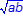• Connection to similar triangles
• Concrete models and exploration activities
• Dynamic geometric software
• Real-world problem situations

Note(s):

• Geometry introduces the geometric mean.
• Previous grade levels solved problems involving similar figures.
• Various mathematical process standards will be applied to this student expectation as appropriate.
• TxCCRS
• III.A. Geometric and Spatial Reasoning – Figures and their properties
• III.A.2. Form and validate conjectures about one-, two-, and three-dimensional figures and their properties.
• III.B. Geometric and Spatial Reasoning – Transformations and symmetry
• III.B.1. Identify transformations and symmetries of figures.
• III.B.2. Use transformations to investigate congruence, similarity, and symmetries of figures.
• III.C. Geometric and Spatial Reasoning – Connections between geometry and other mathematical content strands
• III.C.1. Make connections between geometry and algebraic equations.
• III.D. Geometric and Spatial Reasoning – Measurements involving geometry and algebra
• III.D.3. Determine indirect measurements of geometric figures using a variety of methods.
• VII.A. Problem Solving and Reasoning – Mathematical problem solving
• VII.A.1. Analyze given information.
• VII.A.3. Determine a solution.
• VIII.A. Communication and Representation – Language, terms, and symbols of mathematics
• VIII.A.3. Use mathematical language for reasoning, problem solving, making connections, and generalizing.
• VIII.C. Communication and Representation – Presentation and representation of mathematical work
• VIII.C.1. Communicate mathematical ideas, reasoning, and their implications using symbols, diagrams, models, graphs, and words.
• IX.B. Connections – Connections of mathematics to nature, real-world situations, and everyday life
• IX.B.1. Use multiple representations to demonstrate links between mathematical and real-world situations.
G.9 Similarity, proof, and trigonometry. The student uses the process skills to understand and apply relationships in right triangles. The student is expected to:
G.9A Determine the lengths of sides and measures of angles in a right triangle by applying the trigonometric ratios sine, cosine, and tangent to solve problems.

Determine

THE LENGTHS OF SIDES AND MEASURES OF ANGLES IN A RIGHT TRIANGLE BY APPLYING THE TRIGONOMETRIC RATIOS SINE, COSINE, AND TANGENT TO SOLVE PROBLEMS

Including, but not limited to:

• Trigonometric ratios – a ratio of the measures of two sides of a right triangle based on their position in relation to an acute angle in the right triangle
• Sine
• Cosine
• Tangent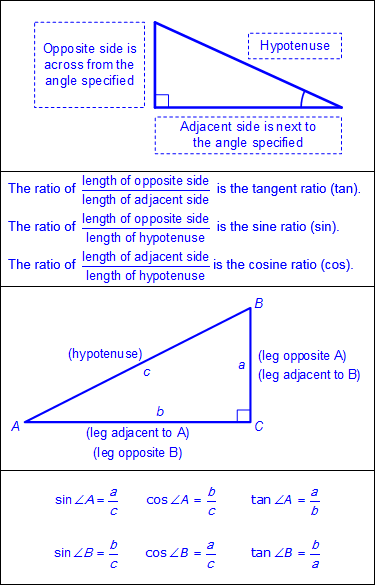• Right triangles
• Side lengths
• Angle measures
• Applications to real-world situations

Note(s):

• Middle School introduced ratios and unit rates when developing proportionality.
• Grade 8 uses the Pythagorean Theorem and its converse to solve problems.
• Geometry introduces trigonometric ratios.
• Various mathematical process standards will be applied to this student expectation as appropriate.
• TxCCRS
• II.A. Algebraic Reasoning – Identifying expressions and equations
• II.A.1. Explain the difference between expressions and equations.
• III.A. Geometric and Spatial Reasoning – Figures and their properties
• III.A.2. Form and validate conjectures about one-, two-, and three-dimensional figures and their properties.
• III.A.3. Recognize and apply right triangle relationships including basic trigonometry.
• III.B. Geometric and Spatial Reasoning – Transformations and symmetry
• III.B.1. Identify transformations and symmetries of figures.
• III.B.2. Use transformations to investigate congruence, similarity, and symmetries of figures.
• III.D. Geometric and Spatial Reasoning – Measurements involving geometry and algebra
• III.D.3. Determine indirect measurements of geometric figures using a variety of methods.
• VII.A. Problem Solving and Reasoning – Mathematical problem solving
• VII.A.1. Analyze given information.
• VII.A.3. Determine a solution.
• VII.B. Problem Solving and Reasoning – Proportional reasoning
• VII.B.1. Use proportional reasoning to solve problems that require fractions, ratios, percentages, decimals, and proportions in a variety of contexts using multiple representations.
• VIII.A. Communication and Representation – Language, terms, and symbols of mathematics
• VIII.A.3. Use mathematical language for reasoning, problem solving, making connections, and generalizing.
• IX.B. Connections – Connections of mathematics to nature, real-world situations, and everyday life
• IX.B.1. Use multiple representations to demonstrate links between mathematical and real-world situations.
G.9B Apply the relationships in special right triangles 30°-60°-90° and 45°-45°-90° and the Pythagorean theorem, including Pythagorean triples, to solve problems.

Apply

THE RELATIONSHIPS IN SPECIAL RIGHT TRIANGLES 30°–60°–90° AND 45°–45°–90° AND THE PYTHAGOREAN THEOREM, INCLUDING PYTHAGOREAN TRIPLES, TO SOLVE PROBLEMS

Including, but not limited to:

• 30°–60°–90° right triangle relationships – the hypotenuse is twice as long as the shorter leg, and the longer leg is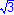times as long as the shorter leg.
• x represents the length of the short leg.
•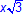represents the length of the longer leg.
• 2x represents the length of the hypotenuse.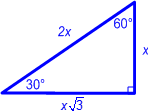• 45°–45°–90° right triangle relationships – the legs are equal in measure and the hypotenuse is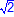times as long as a leg.
• x represents both congruent legs.
•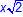represents the hypotenuse.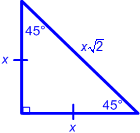• Pythagorean Theorem
• Pythagorean triples – three positive integers, a, b and c, such that a2 + b2 = c2, triples can be generated by multiplying a given Pythagorean triple by any positive integer
• Methods for finding Pythagorean triples
• Beginning with an odd integer
• Let a = an odd integer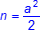b = integer to the left of n on the number line
c = integer to the right of n on the number line
• Beginning with an even integer
• Let a = an even integer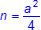b = integer to the left of n on the number line
c = integer to the right of n on the number line
• Solutions with rounded decimal answers
• Applications to real-world situations

Note(s):

• Grade 8 used the Pythagorean Theorem and its converse to solve problems.
• Geometry introduces special right triangles.
• Various mathematical process standards will be applied to this student expectation as appropriate.
• TxCCRS
• II.A. Algebraic Reasoning – Identifying expressions and equations
• II.A.1. Explain the difference between expressions and equations.
• III.A. Geometric and Spatial Reasoning – Figures and their properties
• III.A.2. Form and validate conjectures about one-, two-, and three-dimensional figures and their properties.
• III.A.3. Recognize and apply right triangle relationships including basic trigonometry.
• III.B. Geometric and Spatial Reasoning – Transformations and symmetry
• III.B.1. Identify transformations and symmetries of figures.
• III.B.2. Use transformations to investigate congruence, similarity, and symmetries of figures.
• III.D. Geometric and Spatial Reasoning – Measurements involving geometry and algebra
• III.D.3. Determine indirect measurements of geometric figures using a variety of methods.
• VII.A. Problem Solving and Reasoning – Mathematical problem solving
• VII.A.1. Analyze given information.
• VII.A.3. Determine a solution.
• VII.B. Problem Solving and Reasoning – Proportional reasoning
• VII.B.1. Use proportional reasoning to solve problems that require fractions, ratios, percentages, decimals, and proportions in a variety of contexts using multiple representations.
• VIII.A. Communication and Representation – Language, terms, and symbols of mathematics
• VIII.A.3. Use mathematical language for reasoning, problem solving, making connections, and generalizing.
• IX.B. Connections – Connections of mathematics to nature, real-world situations, and everyday life
• IX.B.1. Use multiple representations to demonstrate links between mathematical and real-world situations.